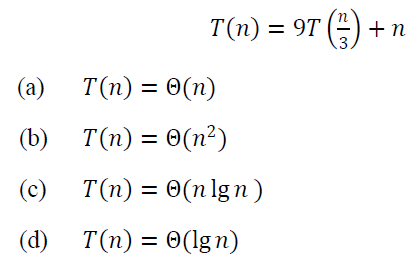1. In a depth-first search of an undirected graph G, every edge of G is:

(a) Either a tree edge or a back edge
(b) Either a forward edge or a cross edge
(c) Either a left edge or a right edge
(d) Either a front edge or a parallel edge

2. The time required to perform a sequence of data structure operations is averaged over all the operations performed is called:

(a) Average case analysis
(b) Amortized analysis
(c) Performance analysis
(d) Best case analysis

3. Which algorithm solves the single-source shortest-paths problem in the general case in which edge weights may be negative?

(a) Dijkstra algorithm
(b) Bellman-Ford algorithm
(c) Ford-Fulkerson algorithm
(d) Prim algorithm

4. Which of the following statements are correct regarding asymptotic notation?

1. 𝑂-notation provides an asymptotic upper bound on a function
2. Ω- notation provides an asymptotic lower bound on a function
3. 𝜃- notation provides an asymptotic lower bound on a function

(a) 1 and 2 only
(b) 1 and 3 only
(c) 2 and 3 only
(d) 1, 2 and 3

5. What is the asymptotic bound for the following recurrence using master theorem method?6. The primary determinant in selecting activities in each iteration of the spiral model of software development is:

(a) Cost
(b) Iteration size
(c) Constraints
(d) Risk

7. Which one of the following testing is essentially a set of path test performed to examine the many different paths through the modules?

(a) Integration testing
(b) Unit testing
(c) Function testing
(d) System testing

8. An approach which is very simple in its philosophy where basically all the modules are constructed and tested independently of each other and when they are finished, they are all put together at same time is:

(a) Top-Down strategy
(b) Bottom-Up strategy
(c) Big-Bang strategy

9. Capability maturity model in software engineering is a technique which is used to improve the:

(a) Testing
(b) Understanding of the software
(c) Software process
(d) Prototype model